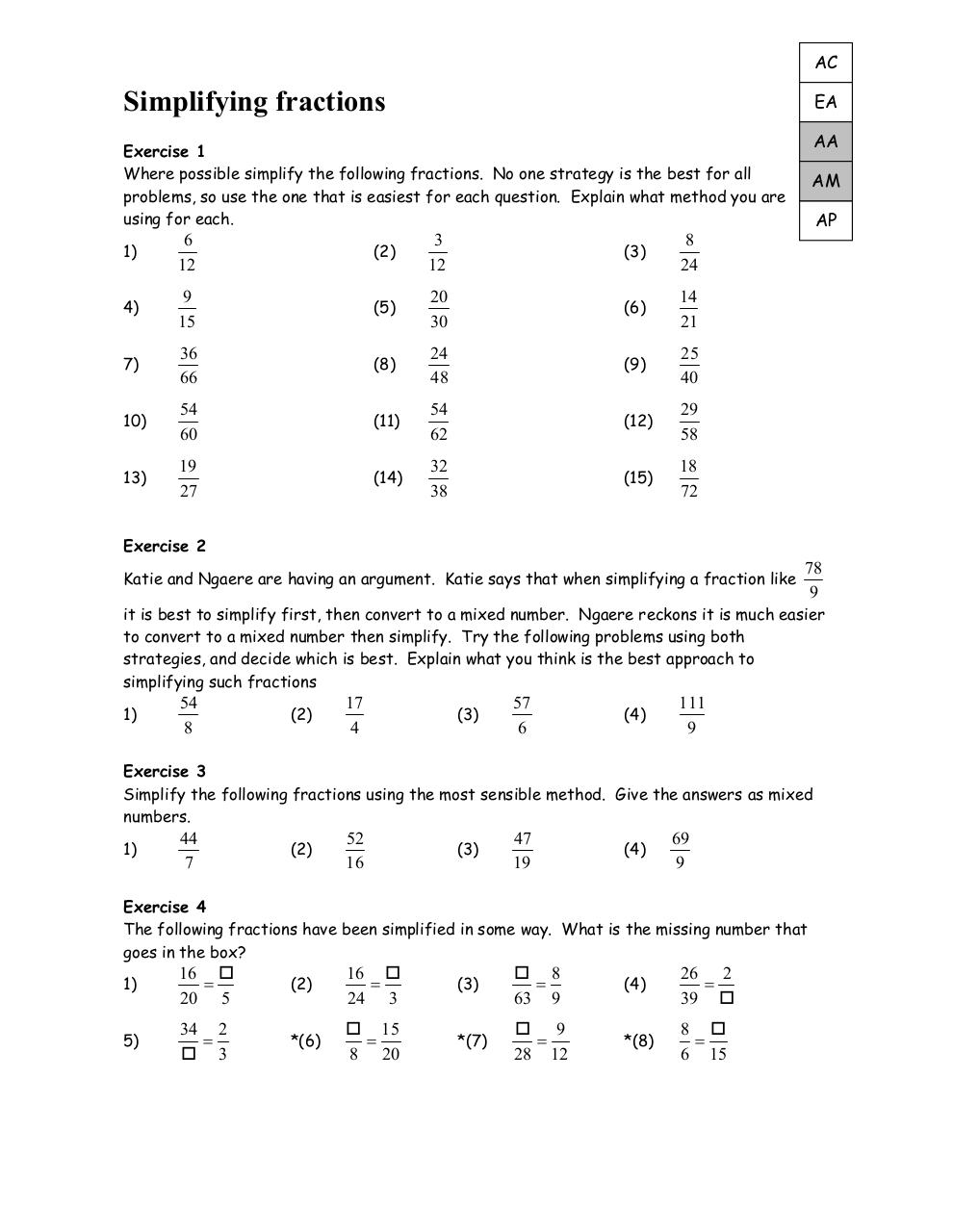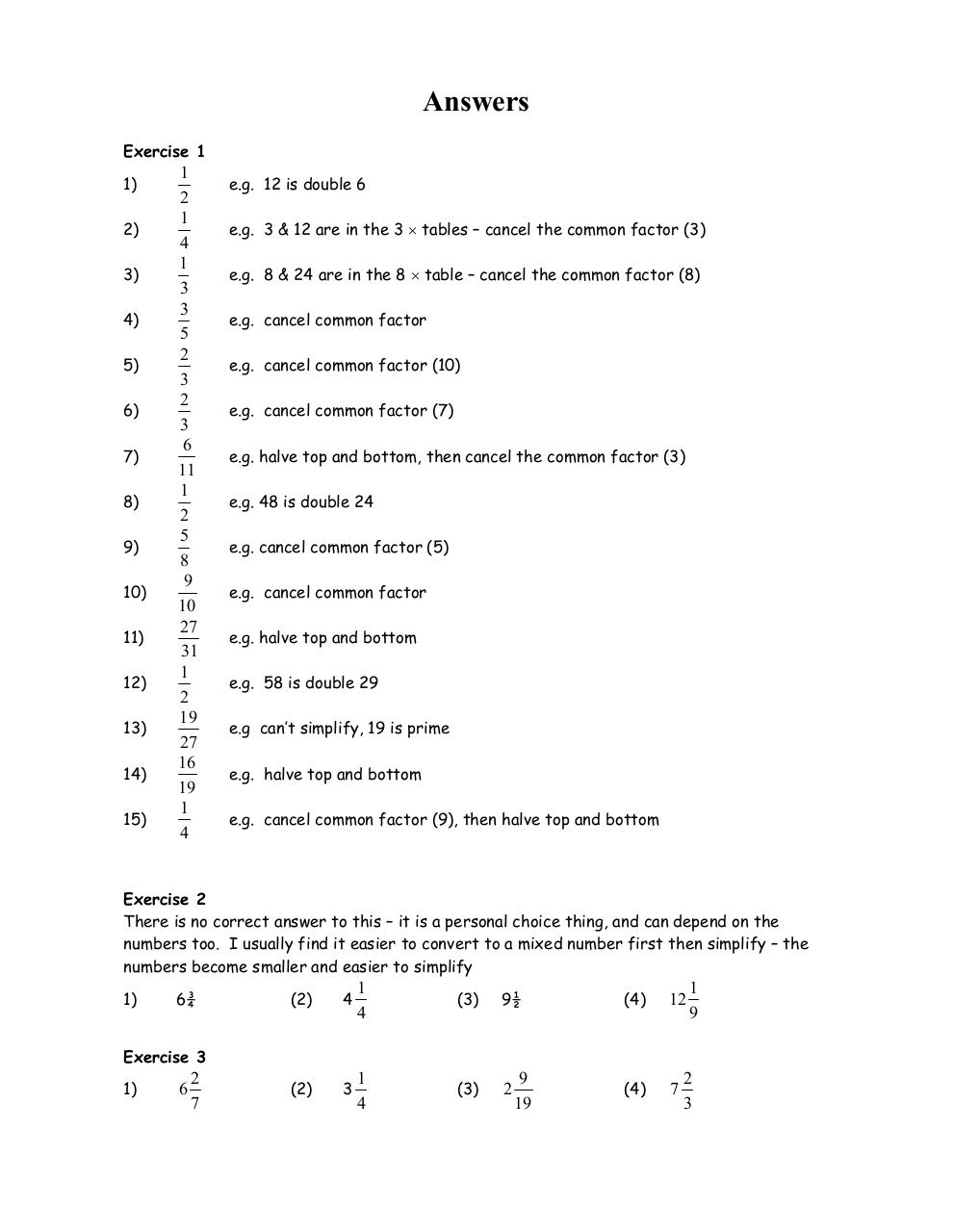# Simplifying Fractions mixed .pdf

### File information

Original filename: Simplifying Fractions mixed.pdf
Title: Microsoft Word - SimplifyingFractions.doc
Author: Andrew Tagg

This PDF 1.3 document has been generated by PScript5.dll Version 5.2.2 / Acrobat Distiller 5.0 (Windows), and has been sent on pdf-archive.com on 22/02/2017 at 06:46, from IP address 78.100.x.x. The current document download page has been viewed 209 times.
File size: 90 KB (3 pages).
Privacy: public file

Simplifying Fractions mixed.pdf (PDF, 90 KB)

### Document preview

AC

Simplifying fractions

EA
AA

Exercise 1
Where possible simplify the following fractions. No one strategy is the best for all
problems, so use the one that is easiest for each question. Explain what method you are
using for each.
1)

6
12

(2)

3
12

(3)

8
24

4)

9
15

(5)

20
30

(6)

14
21

7)

36
66

(8)

24
48

(9)

25
40

10)

54
60

(11)

54
62

(12)

29
58

13)

19
27

(14)

32
38

(15)

18
72

AM
AP

Exercise 2
Katie and Ngaere are having an argument. Katie says that when simplifying a fraction like

78
9

it is best to simplify first, then convert to a mixed number. Ngaere reckons it is much easier
to convert to a mixed number then simplify. Try the following problems using both
strategies, and decide which is best. Explain what you think is the best approach to
simplifying such fractions
1)

54
8

(2)

17
4

(3)

57
6

(4)

111
9

Exercise 3
Simplify the following fractions using the most sensible method. Give the answers as mixed
numbers.
1)

44
7

(2)

52
16

(3)

47
19

(4)

69
9

Exercise 4
The following fractions have been simplified in some way. What is the missing number that
goes in the box?
1)

16 
=
20 5

(2)

16 
=
24 3

(3)

 8
=
63 9

(4)

26 2
=
39 

5)

34 2
=
 3

*(6)

 15
=
8 20

*(7)

 9
=
28 12

*(8)

8 
=
6 15

Exercise 1
1)
2)
3)
4)
5)
6)
7)
8)
9)
10)
11)
12)
13)
14)
15)

1
2
1
4
1
3
3
5
2
3
2
3
6
11
1
2
5
8
9
10
27
31
1
2
19
27
16
19
1
4

e.g. 12 is double 6
e.g. 3 &amp; 12 are in the 3 × tables – cancel the common factor (3)
e.g. 8 &amp; 24 are in the 8 × table – cancel the common factor (8)
e.g. cancel common factor
e.g. cancel common factor (10)
e.g. cancel common factor (7)
e.g. halve top and bottom, then cancel the common factor (3)
e.g. 48 is double 24
e.g. cancel common factor (5)
e.g. cancel common factor
e.g. halve top and bottom
e.g. 58 is double 29
e.g can’t simplify, 19 is prime
e.g. halve top and bottom
e.g. cancel common factor (9), then halve top and bottom

Exercise 2
There is no correct answer to this – it is a personal choice thing, and can depend on the
numbers too. I usually find it easier to convert to a mixed number first then simplify – the
numbers become smaller and easier to simplify
1)

(2)

4

1
4

(3)

(2)

3

1
4

(3)

2

(4)

12

(4)

7

1
9

Exercise 3
1)

6

2
7

9
19

2
3

Exercise 4
1)
=4

(2)

=2

(3)

 = 56

(4)

=3

5)

(6)

=6

(7)

 = 21

(8)

 = 20

 = 51#### HTML Code

Copy the following HTML code to share your document on a Website or Blog

#### QR Code### Related keywords